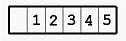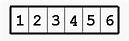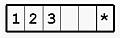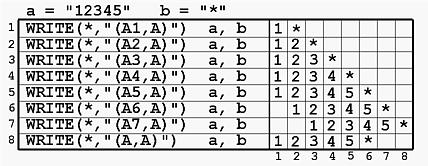# CHARACTER Output: The A DescriptorThe A and Aw descriptors are for CHARACTER output. The general form of these descriptors are as follows:

rA    and    rAw

The meaning of r and w are:

• A is for CHARACTER
• w is the width of field, which indicates that a character string should be printed with w positions.
• The output of the character string depends on two factors, namely the length of the character string and the value of w. Here are the rules:
• If w is larger than the length of the character string, all characters of the string can be printed and are right-justified. Also, leading spaces will be added. The following example prints the string "12345" of length 5 (i.e., five characters) using A6.
```WRITE(*,'(A6)')  "12345"
```
Since w is larger than the length of the string, all five characters are printed and right-justified. The result is shown below:• If w is less than the length of the character string, then the string is truncated and only the left-most w positions are printed in the w positions. The following example prints the string "12345678" of length 8 (i.e., eight characters) using A6.
```WRITE(*,'(A6)')  "12345678"
```
Since w is less than the length of the string, only the first six characters are printed. The result is shown below:• If w is equal to the length of the character string, then it is an exact match. All characters can be printed and all w positions are filled.
• If w is missing (i.e., edit descriptor A), then the value of w is assumed to be the length of the string. The following example shows two WRITE statements. The first one uses A to print a, a string of length 5. The second one also uses A to print b, a string of length 2.
```CHARACTER(LEN=5) :: a = "abcde"
CHARACTER(LEN=2) :: b = "MI"

WRITE(*,'(A)')  a
WRITE(*,'(A)')  b
```
Since the A edit descriptor will use the length of the string as the value of w, the above is equivalent to the following:
```CHARACTER(LEN=5) :: a = "abcde"
CHARACTER(LEN=2) :: b = "MI"

WRITE(*,'(A5)')  a
WRITE(*,'(A2)')  b
```
Therefore, the A edit descriptor is more convenient than Aw.
• r is the repetition indicator, which gives the number of times the edit descriptor should be repeated. For example, 3A5 is equivalent to A5, A5, A5, and 3A is equivalent to A, A, A.
• Please keep in mind that the length of the string includes trailing spaces. For example, if we have the following:
```CHARACTER(LEN=5) :: a = "123"
CHARACTER        :: b = "*"

WRITE(*,"(A,A)")  a, b
```
it is equivalent to
```CHARACTER(LEN=5) :: a = "123"
CHARACTER        :: b = "*"

WRITE(*,"(A5,A1)")  a, b
```
and the result isThe first string, a, is of length 5 and hence the first five positions are used. Why are two extra spaces when the string is written as a = "123"? Since the length of a is five and "123" has only three characters, two spaces are added to the right end to make up five characters. Therefore, variable a actually contains five characters: 1, 2, 3 and two spaces. This is why two spaces are between 123 and * in the above output.### Examples

Let us look at the following example.
```CHARACTER(LEN=5) :: a = "12345"
CHARACTER        :: b = "*"
```
In the following table, the WRITE statements are shown at the left and their corresponding output are shown at the right.• In WRITE statements 1 to 5, since the first A edit descriptor is less than or equal to the length of the character variable, the strings to be printed are truncated from the right.
• In WRITE statements 6 and 7, since the first A edit descriptor is greater than the length of the character variable, leading spaces are added.
• Finally, in the eighth WRITE statement, since the first A has no width of field, it is assumed to be equal to the length of the variable. As a result, A5 is used and the result is identical to that of the fifth WRITE.